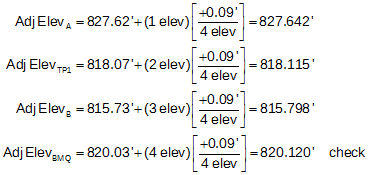### 2. Example 1

A level network, Figure E-2, starts at BM Q, whose elevation is 821.12 ft, travels to points A, TP1, B, and closes back on BM Q. The raw field elevations are shown on the diagram; red numbers are the total of the BS and FS distances between points.Figure E-2 Example Network

Adjust the network using the Equal and Proportional distributions; compare their results.

Using Equation D-4, the network misclosure is: M = 820.03 ft - 820.12 ft = -0.09 ft

#### a. Equal distribution

The Equal distribution applies an equal part of the misclosure to each raw elevation. It is a cumulative correction since each corrected elevation affects following raw elevations by a like amount.Equation E-1 M: misclosure n: number of raw elevations determined i: elevation number

Four raw elevations were established counting the closing elevation on BM Q. Substituting this network's values in Equation E-1:“i” is the seqential number of each elevation in the order they were created; A is 1, TP1 is 2, etc.
The adjusted elevations (computed to an additional decimal place minimizing rounding error) are:#### b. Proportional distribution

The Proportional distribution applies a part of the misclosure to each raw elevation based on sight distances. It is also a cumulative correction since each corrected elevation affects following raw elevations by a like amount.Equation E-2 M: misclosure D: total BS and FS distances d: cumulative distance to current point

The total distance, D, around the loop is 75'+300'+175'+550' = 1100'. Setting up Equation E-2 for this network:The adjusted elevations (computed to an additional decimal place minimizing rounding error) are:#### c. Comparison

 Table E-1 Point Equal Proportional A 827.642 827.626 TP1 818.115 818.101 B 815.798 815.775 BM Q 820.120 820.120

##### (1) Math check

Computing back into BM Q for both methods provides a math check. A common mistake is to forget to reverse the sign of the misclosure. If that were the case, the adjusted elevation at BM Q would be 0.18' too low - twice what it was indicating corrections were applied in the wrong direction.

##### (2) Skipping points

Although the adjusted elevation of TP1 was computed in both methods, it can be skipped since it's only a temporary point. However, any elevation determined in the field, temporary or permanent, must be included in the count (n) for the Equal distribution and the distances through them for the Proportional distribution.

##### (3) Same results?

If the sight distances between points are all equal then the Proportional distribution will result in the same adjusted elevations as the Equal distribution.

##### (4) Random errors only

Misclosure is a result of random errors - mistakes must removed and systematic errors compensated. If they aren’t, then the quality of the adjusted elevations will be degraded. For example, assume the same network but the closing elevation for BM Q was 825.10', 4.98' too high, Figure E-3. A misclosure that large is usually the result of one or more mistakes.Figure E-3 Network with a Mistake

Using an Equal distribution, the equation set up is:If the error occurred between TP1 and B, the raw elevations of A and TP1 won’t be affected by it. Nor will the raw elevation difference between B and BM Q.

If the network is adjusted without first eliminating the mistake, then parts of it will be pushed into all raw elevations. Instead of isolating the error, its effect is spread through the entire network.

If the misclosure is larger than reasonable, look for mistakes or unresolved systematic errors. If neither can be found, or the misclosure is still unreasonably large, then part or all of the network may need to be re-run.